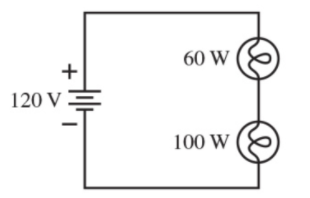# Problem: A 60 W lightbulb and a 100 W lightbulb are placed in the circuit shown in (Figure 1). Both bulbs are glowing. Part A. Which bulb is brighter?A. The 60 W bulb is brighter.B. The 100 W bulb is brighter. C. Both bulbs are equally bright.Part B. Calculate the power dissipated by the 60 W bulb. Express your answer with the appropriate units. Part C. Calculate the power dissipated by the 100 W bulb. Express your answer with the appropriate units.

###### FREE Expert Solution

The power dissipated by a resistor:

$\overline{){\mathbf{P}}{\mathbf{=}}{{\mathbf{i}}}^{{\mathbf{2}}}{\mathbf{R}}}$, where i is the current through the resistor and R is the resistance.

85% (400 ratings)###### Problem Details

A 60 W lightbulb and a 100 W lightbulb are placed in the circuit shown in (Figure 1). Both bulbs are glowing.Part A. Which bulb is brighter?

A. The 60 W bulb is brighter.

B. The 100 W bulb is brighter.

C. Both bulbs are equally bright.

Part B. Calculate the power dissipated by the 60 W bulb. Express your answer with the appropriate units.

Part C. Calculate the power dissipated by the 100 W bulb. Express your answer with the appropriate units.